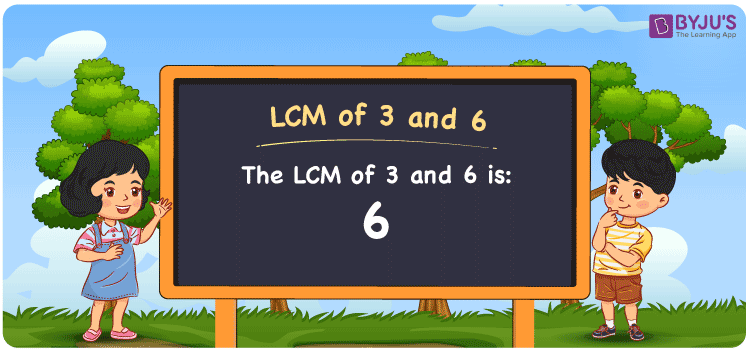Checkout JEE MAINS 2022 Question Paper Analysis : Checkout JEE MAINS 2022 Question Paper Analysis :

# LCM of 3 and 6

LCM of 3 and 6 is 6. The LCM is the value which is divisible evenly by the given two numbers. Least common multiple of 3 and 6 can be obtained from the common multiples of two numbers. (3, 6, 9, 12, 15, 18, 21, ….) and (6, 12, 18, 24, 30, 36, ….) are the multiples of 3 and 6. The LCM of two numbers can be determined by listing the multiples, prime factorization and by division method.

## What is LCM of 3 and 6?

The answer to this question is 6. The LCM of 3 and 6 using various methods is shown in this article for your reference. The LCM of two non-zero integers, 3 and 6, is the smallest positive integer 6 which is divisible by both 3 and 6 with no remainder.## How to Find LCM of 3 and 6?

LCM of 3 and 6 can be found using three methods:

• Prime Factorisation
• Division method
• Listing the multiples

### LCM of 3 and 6 Using Prime Factorisation Method

The prime factorisation of 3 and 6, respectively, is given by:

3 = 3¹

6 = 2 x 3 = 2¹ x 3¹

LCM (3, 6) = 6

### LCM of 3 and 6 Using Division Method

We’ll divide the numbers (3, 6) by their prime factors to get the LCM of 3 and 6 using the division method (preferably common). The LCM of 3 and 6 is calculated by multiplying these divisors.

 2 3 6 3 3 3 1 1

No further division can be done.

Hence, LCM (3, 6) = 6

### LCM of 3 and 6 Using Listing the Multiples

To calculate the LCM of 3 and 6 by listing out the common multiples, list the multiples as shown below

 Multiples of 3 Multiples of 6 3 6 6 12 9 18 12 24

LCM (3, 6) = 6

## Video Lesson on Applications of LCM## LCM of 3 and 6 Solved Example

Question: What is the smallest number exactly divisible by 3 and 6?

Solution:

We know that

LCM is the smallest number exactly divisible by 3 and 6.

Multiples of 3 = 3, 6, 9, 12, 15, ….

Multiples of 6 = 6, 12, 18, 24, 30, …..

Hence, the LCM of 3 and 6 is 6.

## Frequently Asked Questions on LCM of 3 and 6

### What are the methods used to find the LCM of 3 and 6?

The methods used to find the LCM of 3 and 6 are Prime Factorisation, Division method and Listing the multiples.

### Using prime factorisation, find the LCM of 3 and 6.

To find the LCM, we first should know the factors

3 = 3

6 = 2 x 3

LCM is the product of prime factors raised to the highest exponent among 3 and 6.

LCM of 3 and 6 = 2¹ x 3¹ = 6

### Find GCF if the LCM of 3 and 6 is 6.

LCM x GCF = 3 x 6

As the LCM = 6

6 x GCF = 18

GCF = 18/6 = 3